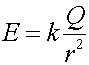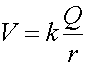Definitions of electric fields and potentials

From Physics 231 and from the previous lecture you should be comfortable with the concepts of force and potential energy. In Physics 231, you used the equation: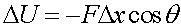where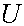is the potential energy added to an object by a force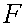pushing it a distance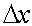. Only the component of force acting parallel to the displacement changes the potential energy; hence the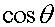factor.

Since all electric forces and potentials are proportional to the charge of an object, it is convenient to define an electric field and electric potential, which are the electric force and the electric potential energy of an object divided by the charge of that object.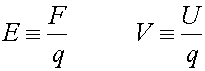The electric field is a vector (it points in the direction of the force that would apply to a positive charge placed in the field) while the electric potential is a scalar (it has no direction).

The magnitude of the electric field, and the value of the electric potential, generated by a point charge are